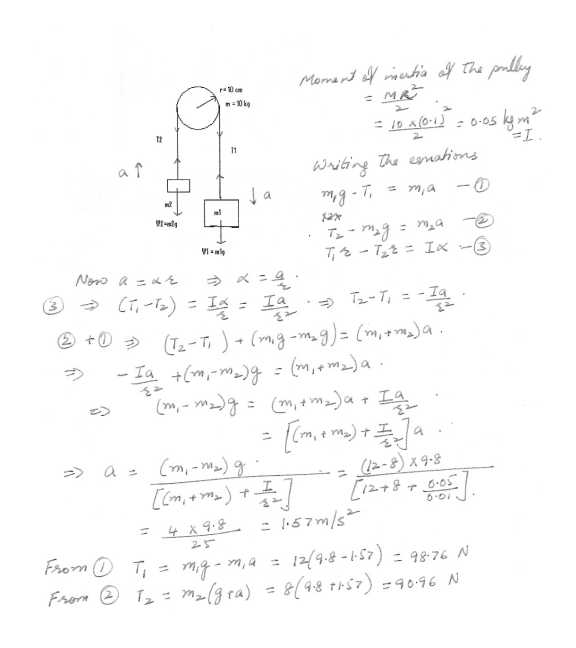41

Physics Force & Laws Of Motion Level: High School

Two masses are suspended from a frictionless pulley as shown in "click here " The pulley itself if has a mass of 10.kg and its radius r = 10 cm.

(a) what is the magnitude of the acceleration of the suspended masses is m1 = 8 kg

(b) what are the tension in the strings? (Neglect the mass of the string.)